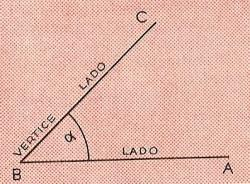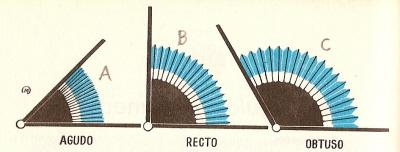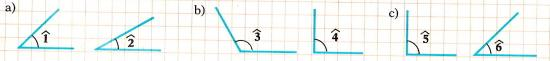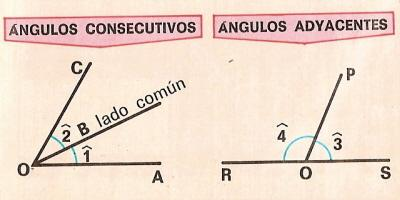Angles ro printName: ______________________________________  Subject: _______________________ Date: _______

Write the missing word

1. Angle. It is the figure formed by two rays, called the sides of the angle.

The angle of the upper drawing has two sides BC and BA.

The origin of the two rays is the vertex B.

This angle is read ABC, mentioning the vertex in the middle.2. The right angle

In the left figure, there are two angles: ABC and CBD, the sum of them is ABD. The two angles are acute.

In the right figure, we see two lines CD and EF intersect at point O. In this case the two lines are perpendicular and form four right angles as the EOD. The acute angle is less than the right angle.

 The angle EOD is... es... The angle ABC is... es... The angle CBD Is... The angle COE is... The angle COF is... The angle ABD is... es... The angle FOD is... es...3. Classes of angles

The angle smaller than the right angle is called acute angle as A, the right angle is formed by two perpendicular lines and is 90 degrees, as B. The obtuse angle is greater than the right, as the C.

Two right angles make a straight angle as D. The reflex angle measures more than 180 degrees, as E and the full angle measures 360 degrees as F.

 The angle B is... The angle A is... The angle C is... The angle F is... The angle D is... The angle E is...Look at the drawing and answer:

 The angle 1 is... The angle 5 is... The angle 2 is... The angle 3 is... The angle 4 is... The angle 6 is...4. Consecutive and adjacent angles

Two consecutive angles are those having the same vertex and a common side between them.

Example: the angles AOB and BOC are consecutive and OB is its common side.

Two angles are adjacent when they are consecutive and its unusual sides are on the same line.

Example: the angles POS and POR are adjacent